# 前言

1、TensorFlow 是由 Google Brain 团队为 深度神经网络（DNN）开发的功能强大的开源软件库；

2、TensorFlow 允许将深度神经网络的计算部署到任意数量的 CPU 或 GPU 的服务器、PC 或移动设备上，且只利用一个 TensorFlow API。

3、那 TensorFlow 与其他深度学习库，如 Torch、Theano、Caffe 和 MxNet的区别在哪里呢？

# TensorFlow程序

``````import tensorflow as tf
message = tf.constant('Hello World!')
with tf.session() as sess:
print(sess.run(message).decode())
``````

tf.session()用法：TensorFlow：tf.Session函数_w3cschool

tf.constant()用法：tf.constant(常量)_bestrivern的博客-CSDN博客_tf.constant

Python decode() 方法：Python decode()方法 | 菜鸟教程

## TensorFlow 程序解读分析

1、 import 模块包含代码将使用的所有库，在目前的代码中只使用 TensorFlow，import tensorflow as tf 则允许 Python 访问 TensorFlow 所有的类、方法和符号。

2、包含 图形定义部分...创建想要的计算图。在本例中计算图只有一个节点，tensor 常量消息由字符串"Welcome to the exciting world of Deep Neural Networks"构成。

3、是通过 会话执行计算图，这部分使用 with 关键字创建了会话，最后在会话中执行以上计算图。

# TensorFlow程序结构（深度剖析）

TensorFlow程序结构（深度剖析）

# TensorFlow常量、变量和占位符详解

TensorFlow常量、变量和占位符详解

1、tensorflow常量

``````#Tensorflow常量举例：
zero_t=tf.zeros([2,3],tf.int32)#创立一个2*3的所有元素都是0的张量
ones_t = tf.ones([2,3],tf.int32)#全部元素都是1
``````

TensorFlow 允许创建具有不同分布的随机张量，也可以随机裁剪张量为指定的大小。

tensorflow参数—seed：如果设置了seed的值，则每次执行程序所产生的随机数或者随机序列均相等，即都为同一个随机数或者随机序列；如果没有设置seed参数的取值，那么每次执行程序所产生的随机数或者随机序列均不等。浅析tensorflow的种子（seed）参数_Legolas~的博客-CSDN博客_tensorflow随机数种子

tensorflow参数—mean：均值（默认为0），stddev：标准差（默认为1.0）。

2、tensorflow变量

``````#两者将被初始化为形状为 [50，50] 的随机均匀分布，最小值=0，最大值=10
rand=tf.random_uniform([50,50],0,10,seed=0)
rand_a=tf.Variable(rand)
rand_b=tf.Variable(rand)

#定义权重和偏置
weight=tf.Variable(tf.random_normal([100,100],stddev=2))
bias=tf.Variable(tf.zeros,name='biases')

#法一：在计算图的定义中通过声明初始化操作对象来实现
sess=tf.session()
init_op=tf.globle_variables_initializer()
sess.run(init_op)

#法二：每个变量也可以在运行图中单独使用 tf.Variable.initializer 来初始化
with tf.session() as sess:
sess.run(bias.initializer)

``````

[En]

The definition of a variable specifies how the variable is initialized, but all declared variables must be explicitly initialized (in calculation or in operation).

3、TensorFlow 占位符—— 它们用于将数据提供给计算图

``````#在这里，为 x 定义一个占位符并计算 y=2*x，使用 feed_dict 输入一个随机的 4×5 矩阵：
x=tf.placeholder("float")
y=x*2
data=tf.random_uniform([4,5],10)
with session() as sess:
x_data=sess.run(data)
print(sess.run(y,feed_dict={x:x_data})
``````

``````t_large=tf.Variable(large_array,trainable=False)
``````

# TensorFlow矩阵基本操作及其实现

``````import tensorflow as tf
#开始一个交互式对话
sess=tf.InteractiveSession()

#创建一个5*5的对角为1单位矩阵
matrix=tf.eye(5)
print(matrix.eval())

#创建一个10*10的对角为1单位矩阵变量
Matrix=tf.Variable(tf.eye(10))
Matrix.initilizer.run()
print(Matrix.eval())

#创建一个5*10的随机矩阵
matrix_t=tf.Variable(tf.random_normal([5,10]))
matrix_t.initilizer.run()

#两个矩阵相乘
product=tf.matmul(matrix_t,Matrix)
print(product.eval())

#类型转换
Matrix_t=tf.Variable(tf.random_uniform([5,10],0,2,dtype=tf.int32))
Matrix_t.initlizer.run()
print(Matrix_t.eval())
Matrix_tnew=tf.cast(Matrix_t,dtype=tf.float32)

#两矩阵相加
print("sum=",sum.eval())

#其他情况
a=tf.Variable(tf.random_normal([4,5],stddev=2))
b=tf.Variable(tf.random_normal([4,5],stddev=2))
A=a*b
B=tf.scalar_mul(2,A)
C=tf.div(a,b)
D=tf.mod(a,b)
init_op=tf.globle_variables_initilizer()
with tf.session() as sess
sess.run(init_op)
write=tf.summary.FileWrite('graphs',sess.graph)
A_,B_,C_,D_=sess.run([A,B,C,D])
print("A=",A_,"\nB=",B_)
writer.close() #需要close才能保存计算图

``````

tf.InteractiveSession()与tf.Session()的区别_XerCis的博客-CSDN博客

Tensorflow.js tf.eye()用法及代码示例 - 纯净天空

tf.random_uniform()_永久的悔的博客-CSDN博客_tf.random_uniform

tf.multiply()、tf.matmul()、tf.scalar_mul()函数介绍和示例_痴迷、淡然~的博客-CSDN博客

TensorFlow四则运算之除法：tf.divide()_凝眸伏笔的博客-CSDN博客_tf.divide

tf.summary.FileWriter用法_小小码农JACK的博客-CSDN博客_tf.summary.filewriter

# TensorFlow TensorBoard可视化数据流—提供计算图的图像

TensorBoard 的 第一步是确定想要的 OP 摘要

``````import tensorflow.compat.v1 as tf

# 定义两个变量
a = tf.placeholder(dtype=tf.float32, shape=[])
b = tf.placeholder(dtype=tf.float32, shape=[])

#添加变量进去，确定想要的摘要
tf.summary.scalar('a', a)
tf.summary.scalar('b', b)

# 将所有summary全部保存到磁盘，以便tensorboard显示
# 摘要将在会话操作中生成,可以在计算图中定义tf.merge_all_summaries OP 一步操作得到摘要，而不需要单独执行每个摘要操作。
smy = tf.summary.merge_all()

#初始化全局变量
init_op = tf.global_variables_initializer()

with tf.Session() as sess:
# 初始化变量
sess.run(init_op)

#把信息存储在具体的文件夹里面
writer = tf.summary.FileWriter("tf_summary_FILE", sess.graph)

#赋值
for i in range(5):
sumers=sess.run(smy,feed_dict={a:i+9,b:i+2})
#把步骤都记录下来

``````

Python—tf.summary.scalar()用法_noedn的博客-CSDN博客_tf.summary.scalar(

``````output_tensor=tf.matmul(input_tensor,weights)+biases
tf.summary.histogram('output',output_tensor)
``````

# TensorFlow指定CPU和GPU设备操作

TensorFlow支持 CPU 和 GPU，也支持分布式计算。可以在一个或多个计算机系统的多个设备上使用 TensorFlow。

TensorFlow 将支持的 CPU 设备命名为"/device：CPU：0"（或"/cpu：0"），第 i 个 GPU 设备命名为"/device：GPU：I"（或"/gpu：I"）。

GPU 比 CPU 要快得多，因为它们有许多小的内核。然而，在所有类型的计算中都使用 GPU 也并不一定都有速度上的优势。为了解决这个问题， TensorFlow 可以选择将计算放在一个特定的设备上。默认情况下，如果 CPU 和 GPU 都存在，TensorFlow 会优先考虑 GPU。

1、 验证 TensorFlow 是否确实在使用指定的设备（CPU 或 GPU），可以创建会话，并将 log_device_placement 标志设置为 True。

``````config=tf.ConfigProto(allow_soft_placement=True,log_device_placement=True)
``````

2、手动选择 CPU 进行操作

``````#手动选择 CPU 进行操作
with tf.device('/cpu:0'):
rand=tf.random_uniform([50,50],0,10,dtype=tf.float32,seed=0)
a=tf.Variable(rand)
b=tf.Variable(rand)
c=tf.matmul(a,b)
init=tf.global_variables_initilizer()

#创建会话
sess=tf.Session(config)
sess.run(init)
print(sess.run(c))
``````

3、手动选择一个 GPU 来操作（同理）

``````with tf.device('/gpu:0'):
rand=tf.random_uniform([50,50],0,10,dtype=tf.float32,seed=0)
a=tf.Variable(rand)
b=tf.Variable(rand)
c=tf.matmul(a,b)
init=tf.global_variables_initilizer()

#创建会话
sess=tf.Session(config=tf.ConfigProto(lod_device_placement=True))
sess.run(init)
print(sess.run(c))
``````

4、手动选择多个GPU

``````c=[]

#多个GPU
for d in ['/gpu:1','/gpu:2']:
with tf.device(d):
rand=tf.random_uniform([50,50],0,10,dtype=tf.float32,seed=0)
a=tf.Variable(rand)
b=tf.Variable(rand)
c.append(tf.matmul(a,b))#在张量的末尾添加新的对象
init=tf.global_variables_initilizer()

#创建会话
sess=tf.Session(config=tf.ConfigProto(allow_soft_placement=True,lod_device_placement=True))
sess.run(init)
print(sess.run(c))
sess.close()

#若系统有3个GPU 设备，那第一组乘法由'/：gpu：1'执行，第二组乘法由'/gpu：2'执行。
``````
• 函数 tf.device() 选择设备（CPU 或 GPU）
• with 块确保设备被选择并用其操作。with 块中定义的变量、常量、操作将用所选择的设备。
• 会话配置使用 tf.ConfigProto 进行控制。通过设置 allow_soft_placement 和 log_device_placement 标志，告诉 TensorFlow 在 指定的设备不可用时自动选择可用的设备，并在 *执行会话时给出日志消息作为描述设备分配的输出，如下图。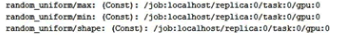# 浅谈深度学习之TensorFlow

1、神经网络是一个生物启发式的计算和学习模型。像生物神经元一样，它们 从其他细胞（神经元或环境）获得加权输入。这个加权输入 经过一个处理单元并产生可以是二进制或连续（概率，预测）的输出。

2、人工神经网络（ANN）是这些神经元的网络，可以 随机分布或排列成一个分层结构。这些神经元通过与它们相关的一 组权重和偏置 来学习

3、深度学习（https://www.cs.toronto.edu/~hinton/absps/NatureDeepReview.pdf）是由 多个处理层（隐藏层）组成的计算模型。层数的增加会导致学习时间的增加。由于数据量庞大，学习时间进一步增加，现今的卷积神经网络（CNN）或生成对抗网络（GAN）的规范也是如此。为了实现 DNN，需要高计算能力。 NVDIA 公司 GPU的问世使其变得可行，随后 Google 的 TensorFlow 使得实现复杂的 DNN 结构成为可能，而不需要深入复杂的数学细节，大数据集的可用性为 DNN 提供了必要的数据来源。

4、任何 深度学习网络都由四个重要部分组成：数据集、定义模型（网络结构）、训练/学习和预测/评估。可以在 TensorFlow 中实现所有这些。

TensorFlow 成为最受欢迎的深度学习库，原因如下：

• TensorFlow 是一个强大的库， 用于执行大规模的数值计算，如矩阵乘法或自动微分。这两个计算是实现和训练 DNN 所必需的。
• TensorFlow 在后端使用 C/C++，这使得计算速度更快。
• TensorFlow 有一个 高级机器学习 API（tf.contrib.learn），可以更容易地配置、训练和评估大量的机器学习模型。
• 可以在 TensorFlow 上使用 高级深度学习库 Keras。Keras 非常便于用户使用，并且可以轻松快速地 进行原型设计。它支持各种 DNN，如RNN、CNN，甚至是两者的组合。

5、数据集

TensorFlow 支持三种主要的读取数据的方法，可以在不同的数据集中使用。

• 通过feed_dict传递数据；
• 从文件中读取数据；
• 使用预加载的数据；

1）通过feed_dict传递数据

``````x=tf.placeholder(tf.float32)
y=tf.placeholder(tf.float32)

with tf.Session as sess:
X_Array=some Numpy Array
Y_Array=some Numpy Array
loss=...#计算loss值

sess.run(loss,feed_dict={x:X_Array,y:Y_Array})
``````

2）通过文件读取

[En]

When the dataset is very large, use this method to ensure that not all data takes up memory immediately.

TensorFlow提供了tf.train.match_filenames_once函数来获取符合一个正则表达式的所有文件，得到的文件列表可以通过tf.train.string_input_producer函数来进行有效管理。

``````#创建文件命名列表
files=tf.train.match_filenames_once('*.JPG')

#创建一个队列来保存文件名
filename_queue=tf.train.string_input_producer(files)

#选择对应的文件阅读器，从文件名队列中读取文件

#使用一个或多个解码器和转换操作,将值字符串解码为训练样本的张量
# record_defaults:指定每一个样本的每一列的类型和默认值
record=[,,] #字符串格式
col1,col2,col3=tf.decode_csv(value,record_defaults=record)
``````

TensorFlow输入文件队列_dz4543的博客-CSDN博客

TensorFlow之数据读取_AI小白一枚的博客-CSDN博客

3）预数据加载

``````#常量
training_data = ...

training_labels = ...

with tf.Session() as sess：
input_data = tf.constant(training_data)
input_labels = tf.constant(training_labels)

#变量
training_data = ...

training_labels = ...

with tf.Session() as sess:
data_initializer = tf.placeholder(dtype=training_data.dtype,shape=training_data.shape)
label_initializer=tf.placeholder(dtype=training_labels.dtype,shape=training_labels.shape)
input_data = tf.Variable(data_initalizer, trainable=False, collections=[])
input_labels = tf.Variable(label_initalizer, trainable=False, collections=[])
...

sess.run(input_data.initializer,feed_dict={data_initializer: training_data})
sess.run(input_labels.initializer,feed_dict={label_initializer: training_lables})
``````

6、定义模型

[En]

A calculation diagram describing the structure of the network is established. It involves * hyperparameters, sequences of variables and placeholders, and loss / error functions that specify information from one group of neurons to another. *

7、训练/学习

8、评估模型

# 常用的三种回归算法

1、线性回归：

• 简单线性回归（一个输入变量，一个输出变量）
• 多元线性回归（多个输入变量，一个输出变量）
• 多变量线性回归（多个输入变量，多个输出变量）

2、逻辑回归：

3、正则化

# TensorFlow损失函数

1、线性回归

``````#定义全局变量
m=1000
n=15
p=2

#标准线性回归
X=tf.placeholder(tf.float32,name='X')#一个输入
Y=tf.placeholder(tf.float32,name='Y')#一个输出
#定义偏置和权重
W0=tf.Variable(0.0)
W1=tf.Variable(0.0)
#线性回归模型
Y_hat=X*W0+W1
#计算loss
loss=tf.square(Y-Y_hat,name='loss')

#多元线性回归
X=tf.placeholder(tf.float32,name='X',shape=[m,n])#多个输入
Y=tf.placeholder(tf.float32,name='Y')#一个输出
W0=tf.Variable(tf.random_normal([n,1]))
W1=tf.Variable(0.0)
Y_hat=tf.matmul(X,W0)+W1
loss=tf.reduce_mean(tf.square(Y-Y_hat),name='loss')
``````

tf.reduce_mean()函数解析（最清晰的解释）_我是管小亮的博客-CSDN博客_tf.reduce_mean

2、逻辑回归

``````X=tf.placeholder(tf.float32,name='X',shape=[m,n])
Y=tf.placeholder(tf.float32,name='Y',shape=[m,p])

W0=tf.Variable(tf.zeros([1,p]),name='bias')
W1=tf.Variable(tf.random_normal([n,1]),name='weights')

Y_hat=dtf.matmul(X*W1)+W0 #[m,p]形式
entropy=tf.nn.softmax_cross_entropy_with_logits(Y_hat,Y) #求单loss
loss=tf.redduce_mean(entropy) #求平均
``````

​​​​​​【TensorFlow】tf.nn.softmax_cross_entropy_with_logits的用法_xf__mao的博客-CSDN博客

【TensorFlow】tf.nn.softmax_cross_entropy_with_logits的用法_zj360202的博客-CSDN博客

tf.nn.softmax参数详解以及作用_dovert的博客-CSDN博客_tf.softmax

3、正则化

``````X=tf.placeholder(tf.float32,name='X',shape=[m,n])
Y=tf.placeholder(tf.float32,name='Y',shape=[m,p])

W0=tf.Variable(tf.zeros([1,p]),name='bias')
W1=tf.Variable(tf.random_normal([n,1]),name='weights')

Y_hat=dtf.matmul(X*W1)+W0 #[m,p]形式
entropy=tf.nn.softmax_cross_entropy_with_logits(Y_hat,Y) #求单loss
loss=tf.reduce_mean(entropy) #求平均

#L1正则化
lamda=tf.constant(0.8)#系数
param=lamda*tf.reduce_sum(tf.abs(W1))#对权重张量的每个元素求和
loss+=prama #new loss

#L2正则化
lamda=tf.constant(0.8)#系数
prama=lamda*tf.nn.l2_loss(W1)#对权重张量的每一个元素平方，然后求和，最后乘1/2
loss+=prama #new loss

``````

tf.nn.l2_loss()的用法_草尖上的舞动的博客-CSDN博客

# TensorFlow优化器使用

[En]

The function reaches its maximum and minimum values where the first derivative is zero. The gradient descent algorithm is based on the same principle, that is, adjusting the coefficient * (weight and bias) to reduce the gradient of the loss function.*

1、首先确定想用的优化器。

``````optimizer=tf.train.GradientDescentOptimizer(learning_rate=0.01)
# learning_rate 参数可以是一个常数或张量,值介于0-1之间。

#必须为优化器给定要优化的函数minimize,实现loss最小化。该方法计算梯度并将梯度应用于系数的学习。
train_step=optimizer.minimize(loss)

with tf.Session() as sess:
sess.run(train_step)
``````

``````optimizer=tf.train.MomentumOptimizer(learning_rate=0.01,momentum=0.5).minimize(loss)
``````

2、衰减学习率

1）变量：

• learning_rate：标量float32或float64张量或者Python数字。初始学习率。
• global_step：标量int32或int64张量或者Python数字。指一次epoch的总共迭代次数，值是等于图像数量/batchsize，用于衰减计算的全局步数，非负。
• decay_steps：标量int32或int64张量或者Python数字。也是指迭代次数，当迭代decay_step次后，改变一次学习率，正数。
• decay_rate：标量float32或float64张量或者Python数字。衰减率。
• staircase：布尔值。若为真则以离散的间隔衰减学习率。
• name：字符串。可选的操作名。默认为ExponentialDecay。

2）返回：

• 与learning_rate类型相同的标量张量，即衰减的学习率。
``````global_step=tf.Variable(0,trainable=false)
initial_learning_rate=0.2
learning_rate=tf.train.exponential_decay(initial_learning_rate,global_step,decay_steps=100000,decay_rate=0.95,staircase=True)
``````

# TensorFlow实现简单线性回归

``````import tensorflow as tf
import numpy as np
import matplotlib.pyplot as plt #绘图

#为了使训练有效，输入应该被归一化，所以定义一个函数来归一化输入数据.

def normalize(X)
mean=np.mean(X)#计算全局平均值
std=np.std(X) #计算全局标准差
X=(X-mean)/std#标准化:对原始数据进行变换到均值为0，标准差为1范围内
return X

#Boston房屋价格数据集中data即为特征变量，target为目标变量。选取data中的RM，target是MEDV变量进行单变量线性回归
#使用TensorFlow contrib数据集加载波士顿房价数据集，并将其分解为 X_train 和 Y_train。可以对数据进行归一化处理：
X_train,Y_train=boston.data[:,5],boston.target #X_train第六列全部数据
X_train=normalize(X_train)
n_sample=len(X_train)

#为训练数据声明 TensorFlow 占位符：
X=tf.placeholder(tf.float32,name='X')
Y=tf.placeholder(tf.float32,name='Y')

#创建 TensorFlow 的权重和偏置变量且初始值为零：
b=tf.Variable(0.0)
w=tf.Variable(0.0)

#定义用于预测的线性回归模型
Y_hat=X*w+b

#定义损失函数
loss=tf.square(Y-Y_hat,name='loss')

#选择梯度优化器
tf.train.GredientDescentOptimizer(learning_rate=0.01).minimize(loss)

#初始化操作符：
init_op=tf.globle_variables_initializer()
total=[]

#计算图
with tf.Session() as sess:
sess.run(init_op)
writer=tf.summary.FileWriter('graphs',sess.graph)
for i in range(100):
total_loss=0
for x,y in zip(X_Train,Y_train)
_,l=sess.run([optimizer,loss],feed_dict={X:x,Y=y}) #返回一次迭代的loss为l
total_loss+=l #total_loss为总的loss值
total.append(total_loss/n_sample) #n_sample为迭代次数，这里默认为len,即batch_size=1
print('Epoch{0}:Loss{1}'.format(i,total_loss/n_sample))
writer.close()
b_value,w_value=sess.run([b,w])

#查看结果
Y_pred=X_train*w_value+b_value
pringt('done')
plt.plot(X_train,Y_train,label='real data')
plt.plot(X_train,Y_pred,label='predict data')
plt.legend()#给图加上图例，没有参数则根据lable自动识别
plt.show()
plt.plot(total)
plt.show()
``````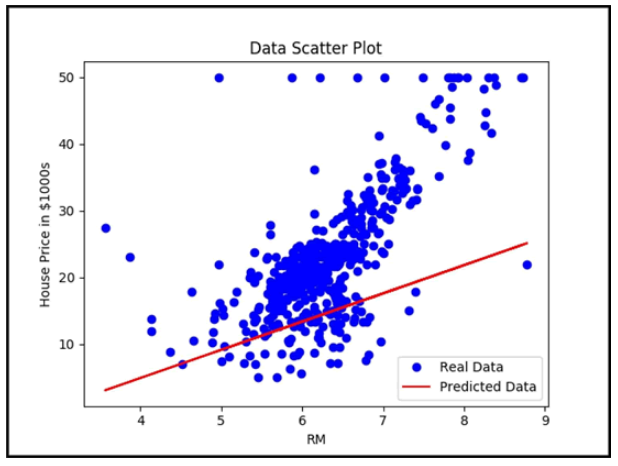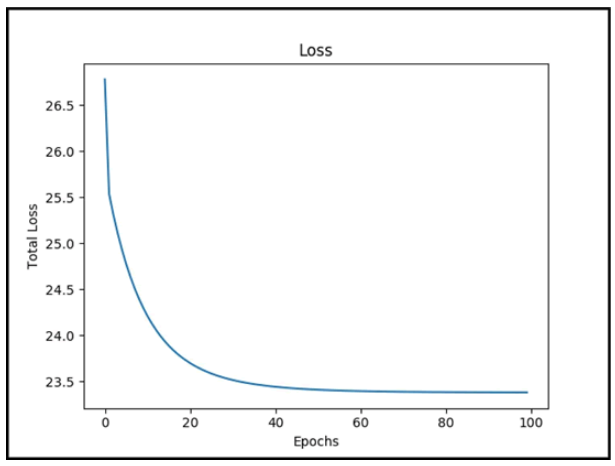2. Python数据可视化matplotlib.pyplot - 简书

data[:,0] data[1,:]的含义_Sophia要一直努力的博客-CSDN博客_data[:]

CUDA、NVIDIA driver、多版本cuda - 知乎

Ubuntu下NVidia驱动、CUDA、CuDNN的安装和卸载 - 知乎

Pandas 库之 DataFrame - 败北桑 - 博客园

Python format 格式化函数 | 菜鸟教程

# TensorFlow实现多元线性回归

``````import tensorflow as tf
import numpy as np
import matplotlib.pyplot as plt #绘图

#为了使训练有效，输入应该被归一化，所以定义一个函数来归一化输入数据.

def normalize(X)
mean=np.mean(X)#计算全局平均值
std=np.std(X) #计算全局标准差
X=(X-mean)/std#标准化:对原始数据进行变换到均值为0，标准差为1范围内
return X

#这里添加一个额外的固定输入值将权重和偏置结合起来。（最好写几个矩阵看一下每行加一然后转置）
def append_bias_reshape(festures,lables):
m=features.shape #获取feature的行数
n=features.sahpe #获取feature的列数
x=np.reshape(np.c_[np.ones(m),features],[m,n+1])#重新组合成新的行列数目
y=np.reshape(lables,[m,1])#重新组合成新的行列数目
return x,y

#注意到X_train包含所需要的特征，可以在这里对数据进行归一化处理，也可以添加偏置并对数据重构：
X_train,Y_train=boston.data,boston.target #全部数据
X_train=normalize(X_train)
X_train,Y_train=append_bias_reshape(X_train,Y_train)
m=len(X_train)#返回列表元素的个数
n=3+1

#为训练数据声明 TensorFlow 占位符。观测占位符 X 的形状变化
X=tf.placeholder(tf.float32,name='X',shape=[m,n])
Y=tf.placeholder(tf.float32,name='Y')

#通过随机数初始化权重：
w=tf.Variable(tf.random_normal([n,1]))

#定义要用于预测的线性回归模型。现在需要矩阵乘法来完成这个任务:
Y_hat=tf.matmul(X,w)

#为了更好地求微分，定义损失函数
loss=tf.reduce_mean(tf.square(Y-Y_hat,name='loss'))

#选择正确的优化器：
tf.train.GredientDescentOptimizer(learing_rate=0.01).minimize(loss)

#初始化操作符：
init_op=tf.globle_variables_initializer()
total=[]

#计算图
with tf.Session() as sess:
sess.run(init_op)
writer=tf.summary.FileWriter('graphs',sess.graph)
for i in range(100):
total_loss=0
for x,y in zip(X_Train,Y_train)
_,l=sess.run([optimizer,loss],feed_dict={X:X_train,Y=Y_train})
total_loss+=l #total_loss为总的loss值
total.append(total_loss/len) #len为一个epoch的总的迭代次数
print('Epoch{0}:Loss{1}'.format(i,total_loss/len))
writer.close()
b_value,w_value=sess.run([b,w])

#绘制损失函数
plt.plot(total)
plt.show()
``````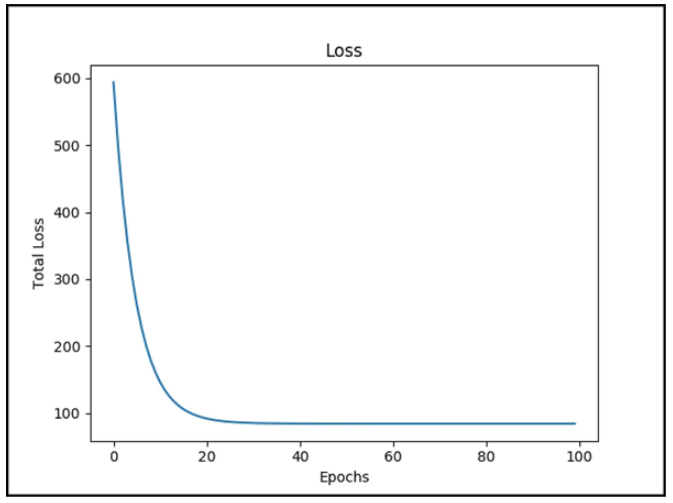numpy np.c_[ ]和np.r_[ ]Cao-Y的博客-CSDN博客_np.r[]

Python中的numpy.ones（）_cunchi4221的博客-CSDN博客

# MNIST数据集简介

MNIST数据集_/home/liupc的博客-CSDN博客_minist数据集

【TensorFlow】MNIST手写数字识别_笔尖bj的博客-CSDN博客

# Tensorflow常用函数

tensorflow笔记 ：常用函数说明_multiangle的博客-CSDN博客

# 机器学习精度评价

TP、True Positive 真阳性：预测为正，实际也为正

FP、False Positive 假阳性：预测为正，实际为负

FN、False Negative 假阴性：预测与负、实际为正

TN、True Negative 真阴性：预测为负、实际也为负。

# Unet模型Unet可以说是 最常用、最简单的一种分割模型了，它简单、高效、易懂、容易构建、可以从小数据集中训练。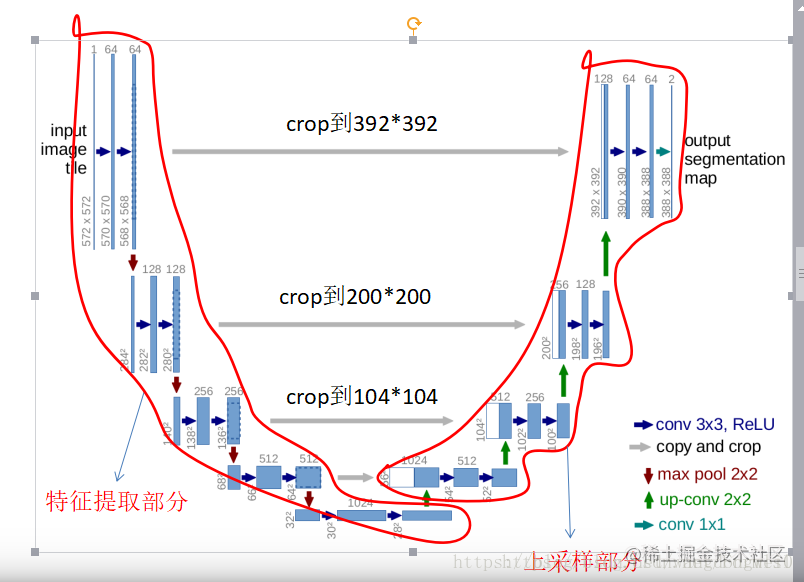• Unet中 卷积池化4次，如开始的图片是224x224的，那就会变成112x112,56x56,28x28,14x14四个不同尺寸的特征。
• 然后我们对14x14的特征图做 上采样或者反卷积，得到28x28的特征图， 这个28x28的特征图与之前的28x28的特征图进行通道伤的拼接concat，然后再对拼接之后的特征图做卷积和上采样，得到56x56的特征图，再与之前的56x56的特征拼接，卷积，再上采样，经过四次上采样可以得到一个与输入图像尺寸相同的224x224的预测结果。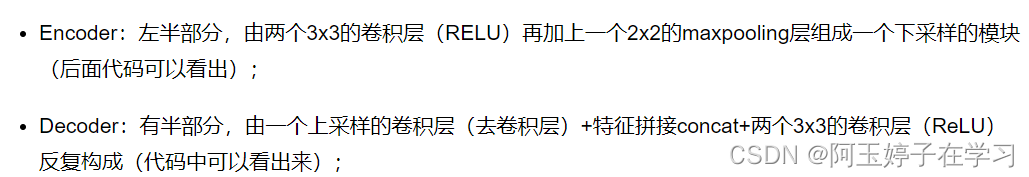# 全卷积网络 FCN 详解

Original: https://blog.csdn.net/weixin_46720482/article/details/124076439
Author: 阿玉婷子在学习
Title: Tensorflow教程

# 学习前言

TF2的也补上咯。其实和Keras的一摸一样0 0。# ; 什么是Focal Loss

Focal Loss是一种Loss计算方案。其具有两个重要的特点。

1、 控制正负样本的权重
2、 控制容易分类和难分类样本的权重

## 一、控制正负样本的权重a的范围是0到1。此时我们便可以通过设置α实现控制正负样本对loss的贡献## ; 二、控制容易分类和难分类样本的权重

Pt的定义如下：( 1 − p t ) γ (1-p_{t})^{γ}(1 −p t ​)γ

1、当pt趋于0的时候，调制系数趋于1，对于总的loss的贡献很大。当pt趋于1的时候，调制系数趋于0，也就是对于总的loss的贡献很小。
2、当γ=0的时候，focal loss就是传统的交叉熵损失，可以通过调整γ实现调制系数的改变。

## 三、两种权重控制方法合并# ; 实现方式

https://github.com/bubbliiiing/yolov4-tf2

location_loss（回归损失）
confidence_loss（目标置信度损失）
class_loss（种类损失）

``````tf.sigmoid(raw_pred[...,4:5])
``````

``````alpha
1-alpha
``````

``````(tf.ones_like(raw_pred[...,4:5]) - tf.sigmoid(raw_pred[...,4:5])) ** gamma
tf.sigmoid(raw_pred[...,4:5]) ** gamma
``````

``````confidence_loss = object_mask * (tf.ones_like(raw_pred[...,4:5]) - tf.sigmoid(raw_pred[...,4:5])) ** gamma * alpha * K.binary_crossentropy(object_mask, raw_pred[...,4:5], from_logits=True) + \
(1 - object_mask) * ignore_mask * tf.sigmoid(raw_pred[...,4:5]) ** gamma * (1 - alpha) * K.binary_crossentropy(object_mask, raw_pred[...,4:5], from_logits=True)
``````

Original: https://blog.csdn.net/weixin_44791964/article/details/123595615
Author: Bubbliiiing
Title: 睿智的目标检测61——Tensorflow2 Focal loss详解与在YoloV4当中的实现

Original: https://blog.csdn.net/weixin_46720482/article/details/124076439
Author: 阿玉婷子在学习
Title: Tensorflow教程

## 一、常见gpu卡计算能力查询

https://developer.nvidia.com/cuda-gpus#compute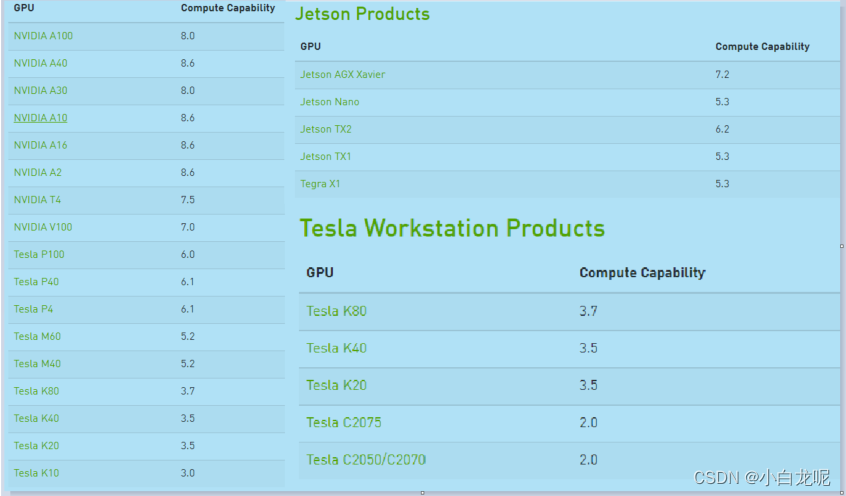## ; 二、计算能力是什么东东

1. 计算能力（Compute Capability） 并不是指gpu的计算性能
2. nvidia发明计算能力这个概念是为了 标识设备的核心架构、gpu硬件支持的功能和指令，因此计算能力也被称为"SM version"。
计算能力包括主修订号X和次修订号Y来表示, 主修订号标明核心架构，次修订号标识在此核心架构上的增量更新。
3. 计算能力版本号与CUDA版本号（例如CUDA7.5、CUDA8等）不能混淆，cuda是一个软件平台，新版本的cuda通过增加默认支持的计算能力进而支持nv新推出的gpu硬件。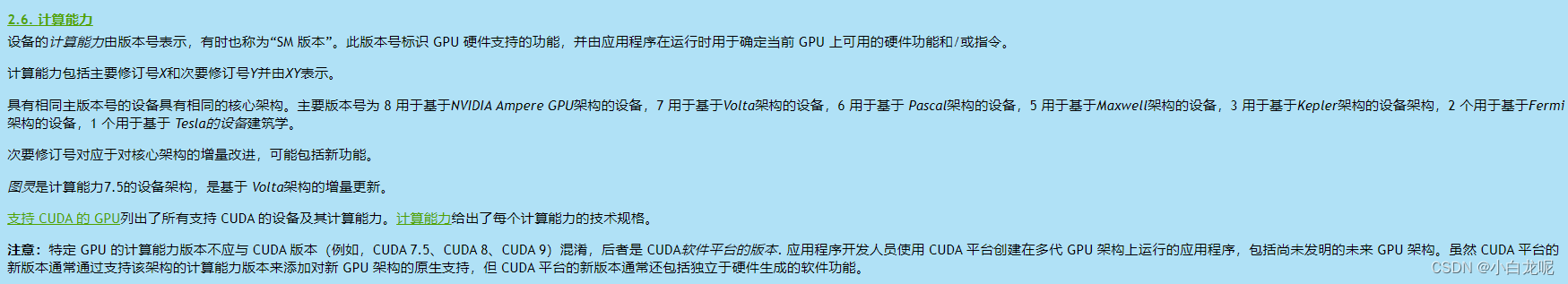## 三、编译常见后端时怎么设置计算能力（亲测有效）

1. 编译tensorflow c++接口设置计算能力
《Tensoflow c++ so编译 基于bazel》 中有说明，不再赘述。
2. 编译pytorch c++接口设置计算能力
pytorch源码：https://github.com/pytorch/pytorch
pytorch在编译过程中使用了环境变量 TORCH_CUDA_ARCH_LIST，在编译前设置此变量即可
例如：
``````export TORCH_CUDA_ARCH_LIST="7.0;7.5;8.6"
python3 ../tools/build_libtorch.py
``````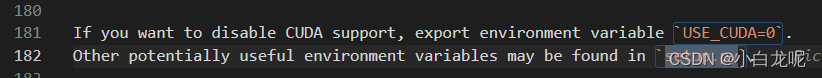1. 编译TensorRT c++接口设置计算能力
增加编译选项 GPU_ARCHS
``````export TRT_RELEASE=/TensorRT-8.2.1.8 #设置TRT未开源包地址，需要提前下载
cmake .. -DTRT_LIB_DIR=\$TRT_RELEASE/lib -DTRT_OUT_DIR=`pwd`/out -DBUILD_PLUGINS=ON -DBUILD_PARSERS=ON -DGPU_ARCHS="70 75 80 86"
make -j
``````
1. 编译caffe c++接口设置计算能力
caffe源码：https://github.com/BVLC/caffe/blob/master/cmake/Cuda.cmake
在caffe 源码目录下的 cmake/Cuda.cmake文件中设置计算能力：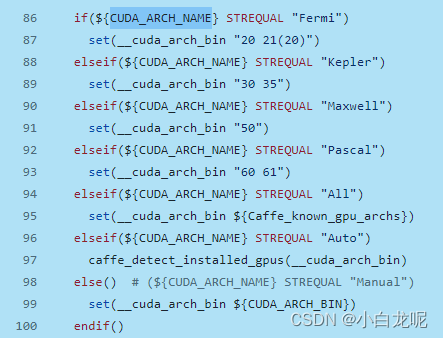``````export CUDA_ARCH_NAME=All

``````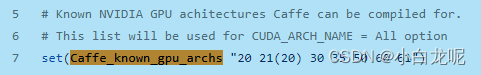## 四、进阶

Original: https://blog.csdn.net/wohenibdxt/article/details/124537949
Author: 小白龙呢
Title: GPU计算能力（Compute Capability）那些事儿

## BIGO | imo实时语音传输优化揭秘

​2020年新冠疫情持续蔓延全球，亿万人的生活因此发生了巨大的改变，人们对实时音视频通话的日常需求也越来越大。作为BIGO公司旗下重要的全球性即时通讯产品imo为全球2亿多用户提供优质稳定的即时通讯服...

## Matlab–k-means聚类算法实现

** Matlab–k-means聚类算法实现 ** 第一次在CSDN写自己的博客，希望开门大吉 哈哈哈 也希望分享的内容大家能够喜欢。 本次创作内容为-----k-means聚类算法实现。 自己用m...

## ResNet网络搭建（tensorflow，keras）

ResNet网络搭建 网络结构及分析： 上述四种CNN，通过加深网络层数，结果会越来越好，但是增大到一定程度，再增加层数，会使神经网络模型退化，因为后面的特征会丢失前面特征的原本模样 因此，将前面的特...